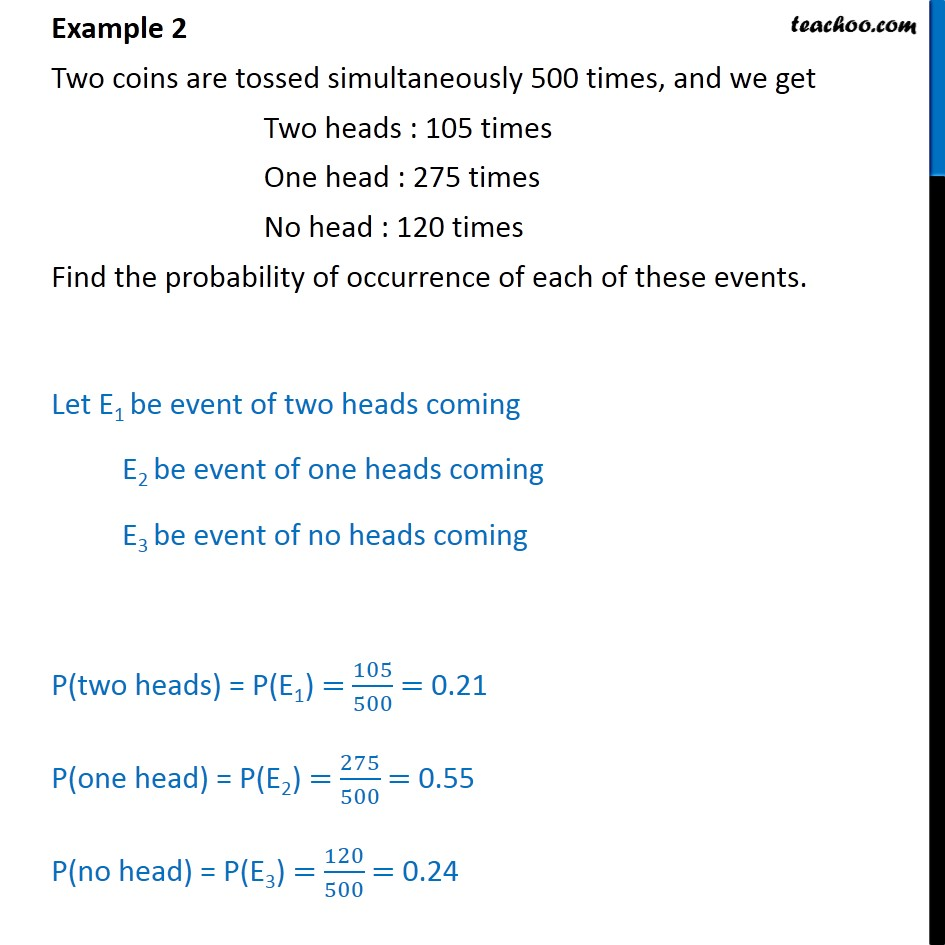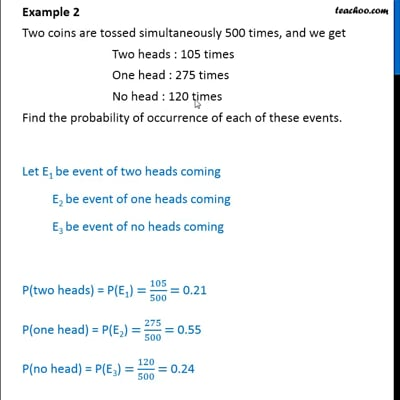Examples

Chapter 15 Class 9 Probability (Term 2)
Serial order wiseThis video is only available for Teachoo black users

### Transcript

Example 2 Two coins are tossed simultaneously 500 times, and we get Two heads : 105 times One head : 275 times No head : 120 times Find the probability of occurrence of each of these events. Let E1 be event of two heads coming E2 be event of one heads coming E3 be event of no heads coming P(two heads) = P(E1) = 105/500 = 0.21 P(one head) = P(E2) = 275/500 = 0.55 P(no head) = P(E3) = 120/500 = 0.24